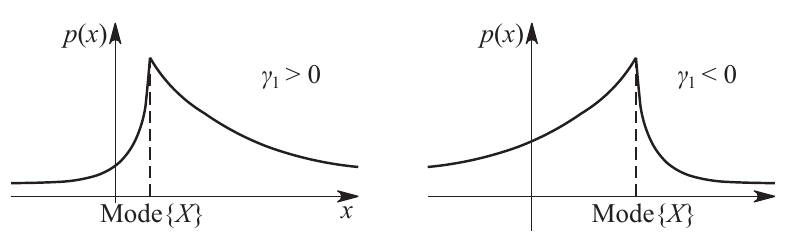This is part of the mo­ments math col­lec­tion.

## Moments

Moments are de­ﬁned for every dis­tri­b­u­tion. These are gen­er­al­ized func­tions which ﬁnd use in a va­ri­ety of sta­tis­ti­cal ex­per­i­men­tal data analy­sis. In par­tic­u­lar, we brieﬂy elu­ci­date how var­i­ous mo­ments are used to char­ac­ter­ize the shape of a dis­tri­b­u­tion.

Finally, we note in pass­ing that a dis­tri­b­u­tion may be to­tally de­scribed via its mo­ments.

Moments

The ex­pec­ta­tion E\{(X-a)^k\} is called the k^{th} mo­ment of the ran­dom vari­able X about the num­ber a

## Initial Moments

Moments about zero are of­ten re­ferred to as the mo­ments of a ran­dom vari­able or the ini­tial mo­ments.

The k^{th} mo­ment sat­is­ﬁes the re­la­tion: \alpha_k=E\{X^k\}= (1)

## Central Moments

When a=E\{X\}, then the k^{th} mo­ment of the ran­dom vari­able X about a is called the k^{th} cen­tral mo­ment.

The k^{th} cen­tral mo­ment sat­is­ﬁes the re­la­tion:

\mu_k=E\{(X-E\{X\})^k\}= (2)

Remark: We note that \mu_0=1 and \mu_1=0 for ran­dom vari­ables.

## Central and Initial MomentRelations

We have:

\mu_k=\sum^k_{m=0} C^m_k\alpha_m(\alpha_1)^{k-m}

\alpha_0=1

\alpha_k=\sum_{m=0}^k C^m_k\mu_m(\alpha_1)^{k-m}

Also we note that, for dis­tri­b­u­tions sym­met­ric about the ex­pec­ta­tion, the ex­ist­ing cen­tral mo­ments \mu_k of even or­der k are zero.

## Condition for UniqueDeterminacy

A prob­a­bil­ity dis­tri­b­u­tion may be uniquely de­ter­mined by the mo­ments \alpha_0,=\alpha_1,\cdot pro­vided that they all ex­ist and the fol­low­ing con­di­tion is sat­is­ﬁed:

\sum_{m=0}^{\infty} |\alpha_m|\frac{t^m}{m!}

## Additional Moments

### Absolute Moments

The k^{th} ab­solute mo­ment of X about a is de­ﬁned by:

m_k=E\{|X-a|^k\}

The ex­is­tence of a k^{th} mo­ment \alpha_k or \mu_k im­plies the ex­is­tence of the mo­ments \alpha_m and \mu_m of all or­ders m<k

### Mixed Moments

We note in pass­ing that the mixed sec­ond mo­ment is bet­ter known as the co­vari­ance of two ran­dom vari­ables and is de­ﬁned as the cen­tral mo­ment of or­der (1+1):

\text{Cov}(X_1,X_2)=\alpha_{1,1}=E\{(X_1-E\{X_1\})(X_2-E\{X_2\})\}

## Moment Interpretations

We note the fol­low­ing:

• The ﬁrst ini­tial mo­ment is the ex­pec­ta­tion.
• The sec­ond cen­tral mo­ment is the vari­ance.
• The third cen­tral mo­ment is re­lated to the skew­ness.
• The fourth cen­tral mo­ment is re­lated to the kur­to­sis.
Skewness

A mea­sure of lop­sid­ed­ness, 0 for sym­met­ric dis­tri­b­u­tions. (a.k.a asym­me­try co­ef­ﬁ­cient)

Mathematically: \gamma_1=\frac{\mu_3}{(\mu_2)^{1.5}}Figure 1: Relationship of the dis­tri­b­u­tion curve and the asym­me­try co­ef­ﬁ­cient.
Kurtosis

A mea­sure of the heav­i­ness of the tail of the dis­tri­b­u­tion, com­pared to the nor­mal dis­tri­b­u­tion of the same vari­ance. (a.k.a ex­cess, ex­cess co­ef­ﬁ­cient) Essentially a mea­sure of the tails of the dis­tri­b­u­tion com­pared with the tails of a Gaussian ran­dom vari­able. (Florescu and Tudor 2013)

Mathematically (Polyanin and Chernoutsan 2010): \gamma_2=\frac{\mu_4}{\mu_2^2}-3

Florescu, I., and C.A. Tudor. 2013. Handbook of Probability. Wiley Handbooks in Applied Statistics. Wiley. https://​books.google.co.in/​books?id=2V3SAQAAQBAJ.

Polyanin, A.D., and A.I. Chernoutsan. 2010. A Concise Handbook of Mathematics, Physics, and Engineering Sciences. CRC Press. https://​books.google.co.in/​books?id=ejzS­cufw­DRUC.